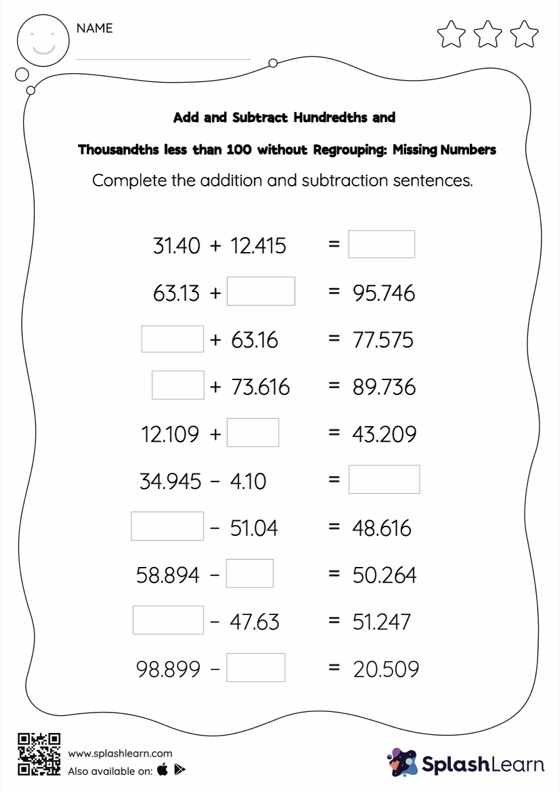# Add and Subtract Hundredths and Thousandths less than 100 without Regrouping: Missing Numbers Worksheet

Home > Add and Subtract Hundredths and Thousandths less than 100 without Regrouping: Missing NumbersStudents find the missing number while adding and subtracting decimals by using the relationship between addition and subtraction. Add and subtract hundredths and thousandths less than 100 without regrouping worksheet does not require them to regroup numbers. This worksheet is about practicing with the horizontal format in which numbers are written side by side. To develop flexibility with numbers and operations, students need to have enough practice in this format and not just rely on the vertical/column method.